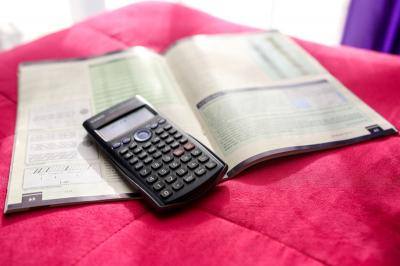• 184
EMPOWERgmat Users
Reported 700+ Last Month
• 770
Top Scorer of the Week
• 440 to 720
Most Improved This Week
(Diag to Final)

### Anatomy of a Quant Question: There’s More Than 1 Way to the Answer

By Max Peterson On Sep 25, 2015 In  Quant Problem Solving Algebra Test The AnswersOne of the great aspects of most of the GMAT Quant questions that you’ll face on Test Day is that they can be approached in more than one way. You can always take a technical approach, but often there is a faster strategic option (one that might require that you get away from the long-winded ‘math’, use the answer choices to your advantage, estimate a solution or even just think logically about the situation). The goal on any Quant question on Test Day is always two-fold:

1 - Get the question correct, if possible.

2 - Answer the question efficiently, without spending too much time on it (so that you have enough time to get to the other questions in the section).

It’s that second ‘piece’ that most Test Takers don’t properly train for. If the approach that you take is the “long way” to get to the answer, then you might find it difficult to finish the section on time and it might be difficult to hit your goal score.

Consider the following Quant question, which involves a fairly standard Algebra concept: a two-variable “system”….

If Jake loses 8 pounds, he will weigh twice as much as his sister. Together they now weigh 278 pounds. What is Jake’s present weight, in pounds?

A – 131

B – 135

C – 139

D – 147

E – 188

Most Test Takers can answer the question without too much trouble, but how much TIME does it take you to answer it? Here are several different ways to approach the question:

1 - Standard Algebra with 2 Variables

There are clearly 2 variables, so we can set up a system of equations…

J = Jake’s present weight

S = his sister’s present weight

(J – 8) = 2(S)

J + S = 278

Since the question asks us for the value of J, we can solve using substitution…

S = 278 – J

(J – 8) = 2(278 – J)

J = 556 – 2J + 8

3J = 564

J = 188

There’s nothing inherently ‘wrong’ with this approach, but it’s arguably the ‘longest’ of the various options.

2 - Standard Algebra with 1 Variable

Jake’s present weight = J

If Jake loses 8 pounds, then he’ll weigh twice his sister’s weight….

Sister’s present weight = (J – 8)/2

Since they weigh a total of 278 pounds….

J + (J – 8)/2 = 278

2J + (J – 8) = 556

3J – 8 = 556

3J = 564

J = 188

This approach, while ‘shorter’ (from a ‘math step’ standpoint), involves a slightly more complex set-up at the beginning.

IF…Jake’s present weight is 147 pounds…and after losing 8 pounds, he will weigh twice as much as his sister….

147 – 8 = 139

139/2 = 69.5

So under these conditions, Jake weighs 147 pounds and his sister weighs 69.5 pounds. That is a total of 147 + 69.5 = 216.5 pounds.

The prompt states that their combined weight is SUPPOSED to be 278 pounds. Since this total is 216.5 pounds, Jake and his sister clearly weigh TOO LITTLE. We need them to both weigh MORE and there’s only one answer that fits…J = 188

This approach has the benefit of giving us real numbers to work with (which, for many Test Takers, is preferable to setting up equations and doing lots of algebra).

4 - Logic

We’re told that the total weight of Jake and his sister = 278 pounds. We’re also told that IF Jake LOST 8 pounds, then he would weight TWICE as much as his sister…

This means that Jake weighs MORE than twice his sister RIGHT NOW. Since the total is 278 pounds, Jake’s weight MUST make up MOST of that total weight (a lot more than half). There’s only one answer that logically fits (2 answers are LESS than half, 1 answer is EXACTLY half and 1 answer is just BARELY MORE than half)… J = 188

This approach takes advantage of the ‘spread’ of the answer choices and the inherent rules behind how the numbers relate to one another. It requires a higher level of attention-to-detail, but less work overall.

The ‘takeaway’ from all of this: just because you get a question correct does not mean that you know everything there is to know about solving it. There could very well be a faster/easier approach. To score at higher levels, you need to know more than one way to get to the correct answer. Learning the necessary Tactics, and practicing them, is essential to your success on Test Day. To that end, we’re here to help.

GMAT assassins aren’t born, they’re made,

Rich

##### Which training plan is best for you?

Get instant advice free. We'll take a look at your target score and your time-frame and recommend the best option for you